# SAT Math Multiple Choice Question 318: Answer and Explanation

### Test Information

Question: 318

3.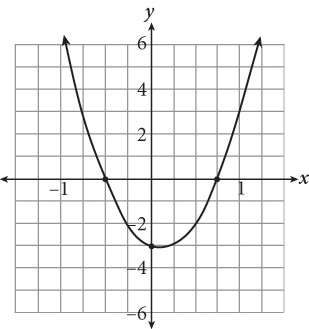Which of the following could be the factored form of the equation graphed in the figure shown?

• A. y = (2x + 1)(4x – 3)
• B. y = (x + 2)(x – 3)
• C.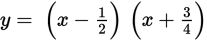• D.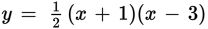Getting to the Answer: An x-intercept is an x-value that corresponds to a y-value of 0. Read the axis labels carefully-each grid-line represents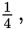so the x-intercepts of the graph, and therefore the roots of the equation, are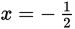and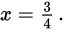This means you are looking for factors that when solved result in these values of x. Choice (A) is correct because 2x + 1 gives youand 4x - 3 gives you